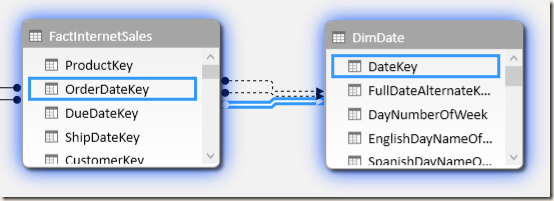## USERELATIONSHIP in a Measure

Consider the classic AdventureWorks data mart example: there are three relationships between the FactInternetSales table and DimDate: one is active (using OrderDateKey) and the other two are inactive (DueDateKey and ShipDateKey).

SalesByOrderDate can be calculated as follows:

`SalesByOrderDate := SUM ( FactInternetSales[SalesAmount] )`

If one wants to use one of the inactive relationships, they just have to apply the USERELATIONSHIP function in one of the CALCULATE filter arguments:

```SalesByDueDate :=
CALCULATE (
SUM ( FactInternetSales[SalesAmount] ),
USERELATIONSHIP (
FactInternetSales[DueDateKey],
DimDate[DateKey]
)
)

SalesByShipDate :=
CALCULATE (
SUM ( FactInternetSales[SalesAmount] ),
USERELATIONSHIP (
FactInternetSales[ShipDateKey],
DimDate[DateKey]
)
)```

The following screenshot displays the different values for the three measures for each year.

## USERELATIONSHIP in a Calculated Column

When one defines a calculated column, they are writing a DAX expression that will be executed in a row context. Since USERELATIONSHIP requires a CALCULATE to be used and the CALCULATE applies a context transition when executed within a row context, obtaining the desired behavior is not easy.

## Applying USERELATIONSHIP to RELATED

If one creates a calculated column in FactInternetSales, one might want to use RELATED choosing the relationship to use. Unfortunately, this is not possible. For example, in order to denormalize the day name of week of the order date, one writes:

```FactInternetSales[DayOrder] =
RELATED ( DimDate[EnglishDayNameOfWeek] )```

But what if the user wants to obtain the day name of week of the due date? They cannot use CALCULATE and RELATED together, so they have to use this syntax instead:

```FactInternetSales[DayDue] =
CALCULATE (
CALCULATE (
VALUES ( DimDate[EnglishDayNameOfWeek] ),
FactInternetSales
),
USERELATIONSHIP ( DimDate[DateKey], FactInternetSales[DueDateKey] ),
ALL ( DimDate )
)```

Two CALCULATE are required in this case: the outermost CALCULATE applies the USERELATIONSHIP to the innermost CALCULATE, and the ALL ( DimDate ) filter removes the existing filter that would be generated by the context transition. The innermost CALCULATE applies FactInternetSales to the filter condition. Thanks to the active USERELATIONSHIP, its filter propagates to the lookup DimDate table using the DueDateKey relationship instead of the OrderDateKey relationship. The result is visible in the following screenshot.

Though this syntax works, it is strongly advised not to use it. Indeed, it is hard to understand and it is easy to write incorrect DAX code here. A better approach is using LOOKUPVALUE instead, which does not require the relationship at all.

```FactInternetSales[DayDue] =
LOOKUPVALUE (
DimDate[EnglishDayNameOfWeek],
DimDate[DateKey],
FactInternetSales[DueDateKey]
)```

Performance data is not available here. However, in a calculated column the difference is not important, meaning as a rule, the clarity of the expression should be favored.

## Applying USERELATIONSHIP to RELATEDTABLE

If RELATEDTABLE is used in a calculated column, one cannot apply USERELATIONSHIP directly. However, this can easily be done by replacing RELATEDTABLE with CALCULATETABLE. As an example, consider the following calculated column in DimDate table:

```DimDate[OrderSales] =
SUMX (
RELATEDTABLE( FactInternetSales ),
FactInternetSales[SalesAmount]
)```

RELATEDTABLE can always be rewritten using CALCULATETABLE.

```DimDate[OrderSales] =
SUMX (
CALCULATETABLE( FactInternetSales ),
FactInternetSales[SalesAmount]
)```

These are in reality the same function, but CALCULATETABLE can accept additional filter arguments. Thus, a DueSales calculated column can be written as follows:

```DimDate[DueSales] =
SUMX (
CALCULATETABLE (
FactInternetSales,
USERELATIONSHIP (
FactInternetSales[DueDateKey],
DimDate[DateKey]
)
),
FactInternetSales[SalesAmount]
)```

The following screenshot displays the result of the two calculated columns:

Thus, using USERELATIONSHIP is possible if using CALCULATETABLE instead of RELATEDTABLE.